Next: C.3 Estimate of error Up: C. Proofs Previous: C.1 Probabilistic PCA and

# C.2 The eigenvalue equation in kernel PCA

The equivalence of the equations

 n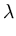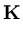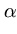=(C.8)

and

 n=(C.9)

is shown.

Equation (C.8) follows immediately from (C.9). To prove the opposite direction, we assume that a vector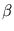exists that is not an eigenvector of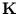, whileis an eigenvector of. This assumption infers that (C.8) is fulfilled and (C.9) is not. Thus, we need to show that the assumption leads to a contradiction.

We only consider the case thatis orthogonal to the subspace ker(the space of vectors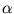fulfilling= 0) because the elements of ker--if they exist--solve already both (C.8) and (C.9). Sinceis symmetric,can be written as a linear combination of the pairwise orthogonal eigenvectorsl of,=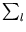ull. At least, two ul must differ from zero becauseitself is not an eigenvector. It follows that=ul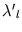l withbeing the eigenvalues corresponding tol. All eigenvalues are non-zero becauseis orthogonal to ker. Thus,can be also not an eigenvector of. This contradicts our first assumption. Therefore, (C.9) follows from (C.8).Next: C.3 Estimate of error Up: C. Proofs Previous: C.1 Probabilistic PCA and
Heiko Hoffmann
2005-03-22